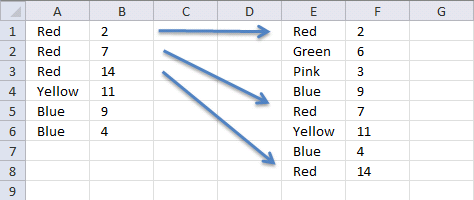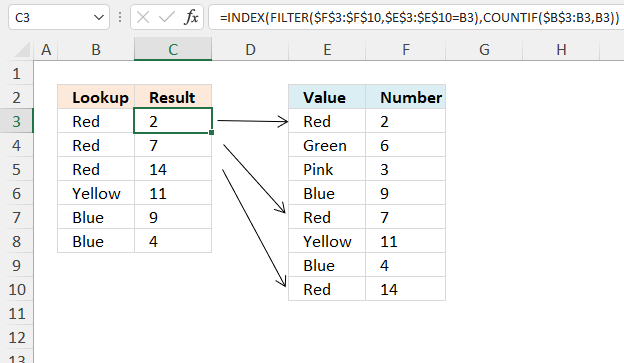Author: Oscar Cronquist Article last updated on August 26, 2022## 1. Vlookup with multiple matches returns a different valueLinda asks in this post: How to return multiple values using vlookup in excel

I tried using the formula above but it didn't work for me and I can't figure out how to adjust it to accomodate my needs.

Here is what I have: Data Range is in \$E\$1:\$F\$8, I would like my results in Col. B. Lookup value in column A and return the value in Col F that matches.

Since there are duplicates in Col. A I want Col. B to return the next matching value from col. F.

Essentially this is a Vlookup with multiple matches that would return a different value. Thanks for any help you can provide.

Data Range Col. A Col B
Red 2 Red
Green 6 Red
Pink 3 Red
Blue 9 Yellow
Red 7 Blue
Yellow 11 Blue
Blue 4
Red 14

Array Formula in cell B1:

=INDEX(\$F\$1:\$F\$8, SMALL(IF(A1=\$E\$1:\$E\$8, ROW(\$E\$1:\$E\$8)-MIN(ROW(\$E\$1:\$E\$8))+1, ""), COUNTIF(A1:\$A\$1, A1)))

How to enter an array formula

1. Select cell B2
2. Type the formula above
3. Press and hold CTRL + SHIFT
4. Press Enter
5. Release all keys

If you did it right, the formula now has curly brackets before and after, like this: {=array_formula}.

Copy cell B1 and paste it down as far as needed.

### Explaining formula in cell B1

#### Step 1 - Find value

A1=\$E\$1:\$E\$8

becomes

="Red={"Red"; "Green"; "Pink"; "Blue"; "Red"; "Yellow"; "Blue"; "Red"}

and returns

{TRUE; FALSE; FALSE; FALSE; TRUE; FALSE; FALSE; TRUE}

#### Step 2 - Replace TRUE with corresponding row number

The IF function has three arguments, the first one must be a logical expression. If the expression evaluates to TRUE then one thing happens (argument 2) and if FALSE another thing happens (argument 3).

IF(A1=\$E\$1:\$E\$8, ROW(\$E\$1:\$E\$8)-MIN(ROW(\$E\$1:\$E\$8))+1, "")

becomes

IF({TRUE; FALSE; FALSE; FALSE; TRUE; FALSE; FALSE; TRUE}, ROW(\$E\$1:\$E\$8)-MIN(ROW(\$E\$1:\$E\$8))+1, "")

becomes

IF({TRUE; FALSE; FALSE; FALSE; TRUE; FALSE; FALSE; TRUE}, {1;2;3;4;5;6;7;8}, "")

and returns

{1;"";"";"";5;"";"";8}

#### Step 3 - Extract k-th smallest row number

To be able to return a new value in a cell each I use the SMALL function to filter row numbers from smallest to largest based on corresponding value.

SMALL(IF(A1=\$E\$1:\$E\$8, ROW(\$E\$1:\$E\$8)-MIN(ROW(\$E\$1:\$E\$8))+1, ""), COUNTIF(A1:\$A\$1, A1))

becomes

SMALL({1;"";"";"";5;"";"";8}, COUNTIF(A1:\$A\$1, A1))

The COUNTIF function counts values based on a condition or criteria, the first argument contains an expanding cell reference, it grows when the cell is copied to cells below. This lets the formula count values.

SMALL({1;"";"";"";5;"";"";8}, 1)

and returns 1.

#### Step 4 - Return value based on row number

The INDEX function returns a value based on a cell reference and column/row numbers.

INDEX(\$F\$1:\$F\$8, SMALL(IF(A1=\$E\$1:\$E\$8, ROW(\$E\$1:\$E\$8)-MIN(ROW(\$E\$1:\$E\$8))+1, ""), COUNTIF(A1:\$A\$1, A1)))

becomes

INDEX(\$F\$1:\$F\$8, 1)

and returns 2 in cell B1.

### Get the Excel fileVlookup-next-matching-item.xls

## 2. Lookup with multiple matches returns different values - Excel 365This example shows a formula that performs a lookup based on the number of instances of a particular condition. This means that the formula returns a different value for each duplicate value corresponding to the data set.

Excel 365 formula in cell C3:

=INDEX(FILTER(\$F\$3:\$F\$10, \$E\$3:\$E\$10=B3), COUNTIF(\$B\$3:B3, B3))

### Explaining formula

#### Step 1 - Logical test

The equal sign is a logical operator that compares value to value, it is not case sensitive. The result is a boolean value TRUE or FALSE if the condition is met or not.

\$E\$3:\$E\$10=B3

becomes

{"Red"; "Green"; "Pink"; "Blue"; "Red"; "Yellow"; "Blue"; "Red"}="Red"

and returns

{TRUE; FALSE; FALSE; FALSE; TRUE; FALSE; FALSE; TRUE}.

#### Step 2 - Extract values based on a condition

The FILTER function extracts values/rows based on a condition or criteria.

Function syntax: FILTER(array, include, [if_empty])

FILTER(\$F\$3:\$F\$10, \$E\$3:\$E\$10=B3)

becomes

FILTER({2; 6; 3; 9; 7; 11; 4; 14}, {TRUE; FALSE; FALSE; FALSE; TRUE; FALSE; FALSE; TRUE})

and returns

{2; 7; 14}.

#### Step 3 - Count value across cells using a dynamic reference

\$B\$3:B3 is both an absolute and relative cell reference, \$B\$3 is absolute and B3 is a relative. This means that the cell reference grows when the cell is copied to cells below, it keeps track of how many instances of the current condition there are based on the cell and also the cells above the condition.

The COUNTIF function calculates the number of cells that is equal to a condition.

Function syntax: COUNTIF(range, criteria)

COUNTIF(\$B\$3:B3, B3)

becomes

COUNTIF("Red", "Red")

and returns 1.

#### Step 4 - Get value

The INDEX function returns a value or reference from a cell range or array, you specify which value based on a row and column number.

Function syntax: INDEX(array, [row_num], [column_num])

INDEX(FILTER(\$F\$3:\$F\$10, \$E\$3:\$E\$10=B3), COUNTIF(\$B\$3:B3, B3))

becomes

INDEX({2; 7; 14}, 1)

and returns 2.